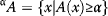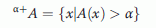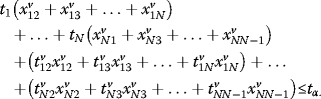## Background

Vehicle routing problems (VRPs) have a significant role in logistics and distribution industries. VRPs include problems in which a fleet of vehicles presents service from one or several depots to different geographically located customers so that the total cost of transportation is minimized. It has been proven that vehicle routing problems are categorized as non-deterministic polynomial (NP)-hard problems ([Lenstra and Rinnooy Kan 1981]; [Mingozzi et al. 1999]) and that exact solution methods are not practical in a large scale due to high computational cost since most of the procedures have focused on using heuristics and meta-heuristics.

This problem was investigated mathematically by ([Dantzig et al. 1994]). The primitive model was developed by other researchers. [Clarke and Wright (1994]) solved VRPs by using a saving algorithm. Developing VRP models improves advanced solving methods based on mathematical programming, heuristics and meta-heuristics. [Altinkemerk (1991]) used a sweeping algorithm to develop a corresponding mathematical model. [Laporte (1992]) and Laporte et al. (1992) propagated a branch-and-bound algorithm. [Tavakkoli-Moghaddam et al. (2005]) proposed a multi-criteria vehicle routing problem considering soft time windows, and their problem is solved by simulated annealing. Also Tavakkoli-Moghaddam et al. ([2006b]) developed a mathematical model for VRP with backhauls by using memetic algorithm. Furthermore, [Tavakkoli-Moghaddam et al. (2006b]) presented a linear integer model of capacitated vehicle routing problems with the independent route length to minimize the heterogeneous fleet cost and maximize the capacity utilization, and their proposed model is solved by a hybrid simulated annealing (SA) based on the nearest neighborhood. [Jozefowiez et al. (2009]) developed a multi-objective vehicle routing problem by minimizing the route length and route balance by using a meta-heuristic method with traditional operators. A number of meta-heuristics, such as SA, tabu search and ant colony system, have been developed to solve more complicated problems containing more constraints ([Fallahi et al. 2008]; [Bin et al. 2009]). In general, the presented models for VRPs consider the cost function and propose the solution based on the cost minimization. However, there are other factors which have significant effect on the problem, such as drivers' satisfaction, which is investigated in this paper.

Since traveling time of the vehicle cannot be determined certainly due to various factors, such as traffic congestion, weather condition, vehicle failure and the like, this parameter is considered by a probability function. Obviously, increasing the total service time leads to decreasing the driver's satisfaction. In this paper, the total cost function of the vehicles is minimized, while the driver's satisfaction is increased by decreasing the probability of the service time. This problem is considered as a graph by n nodes, which represent customers, and connecting arcs show the route between customers. Each customer has a specific demand, and they should be served by only one vehicle. Due to its successful result in meta-heuristics, the model is solved by a hybrid simulated annealing (HSA) algorithm ([Tavakkoli-Moghaddam et al. 2006a]). The proposed algorithm shows much higher efficiency than the Lingo 8 software in large scale problems.

The rest of this paper is organized as follows. Problem definition introduces the problem, and Model formulation represents the model formulation. Problem-solving methodology is described in Hybrid simulation annealing, and computational results are discussed in Results and discussion. Finally, a conclusion follows in the last section.

## Methods

### Problem definition

The traveling time for vehicles is a probability variable depending on the weather conditions, accident, failure of the vehicle and condition of the route. Before describing the problem, two definitions are presented based on the fuzzy theory ([Zimmermann 1993]).

#### Fuzzy number

Definition 2.1. A fuzzy set A in R (real line) is defined to be a set of ordered pairs $A=\left\{\left(x,{\phantom{\rule{0.1em}{0ex}}}_{\mu }\left(x\right)\right)|xR\right\}$, where A (x) is called the membership function for the fuzzy set.

Definition 2.2. A fuzzy set A is called normal if there is at least one point $xR$ with A (x) = 1.

Definition 2.3. A fuzzy set A on R is convex if, for any x,y R and any $\left[0,1\right]$, we have ${\phantom{\rule{0.1em}{0ex}}}_{A}\left(x+\left(1\right)y\right)min\left\{{\phantom{\rule{0.1em}{0ex}}}_{A}\left(x\right),{\phantom{\rule{0.1em}{0ex}}}_{A}\left(y\right)\right\}$.

Definition 2.4. A fuzzy number is a fuzzy set on the real line that satisfies the conditions of normality and convexity.

#### α-cut

One of the most important concepts of fuzzy sets is -cut. Given fuzzy set A defined on X and any number $\left[0,1\right]$, the -cut (i.e. A) and the strong -cut (i.e. +A) are the crisp sets.(1)(2)

Also, distributing companies are interested in the service time of each vehicle not exceeding from a specific time limit. Increasing of the service time leads to decreasing of the driver's working utility. It is supposed that the maximum time working utility of the drivers is achieved when service time is less than t l in bound of [0, t u ] and that increasing the service time affects linearly on the decreasing of the utility so that, for service time more than t u , the utility level achieves its minimum. Also, a driver's utility function versus time is illustrated by a fuzzy number as shown in Figure 1. This function states that the driver's utility decreases linearly as traveling time increases.

Assumptions used in our presented model are as follows:

1. 1.

Travel time of each route is a normal variable with the specific mean and specific variance.

2. 2.

The driver's utility function depends on the travel time, and it decreases linearly with increasing the travel time.

In this way, a stochastic model is presented such that a vehicle travel time is restricted to time less than t with p percent probability in each level of satisfaction. t is the maximum length of tour in time for level of satisfaction. To develop this model, the sum of the normal distribution function theorem is described in this section. Based on this theorem, if x i is an independent variable of the normal distribution with meani's and variancei's by ${x}_{i}N\left({\phantom{\rule{0.1em}{0ex}}}_{i},{i}^{2}\right)$, in which a i is the constant number, y is a summation of x i 's by:

$y={a}_{1}{x}_{1}+{a}_{2}{x}_{2}++{a}_{n}{x}_{n}$

Then, y is also a normally distributed variable given by $yN\left({\phantom{\rule{0.1em}{0ex}}}_{y},{\phantom{\rule{0.1em}{0ex}}}_{y}^{2}\right)$, where ${\phantom{\rule{0.1em}{0ex}}}_{y}={a}_{1}{\phantom{\rule{0.1em}{0ex}}}_{1}+{a}_{2}{\phantom{\rule{0.1em}{0ex}}}_{2}++{a}_{n}{\phantom{\rule{0.1em}{0ex}}}_{n}$ and ${\phantom{\rule{0.1em}{0ex}}}_{y}^{2}={a}_{1}^{2}{\phantom{\rule{0.1em}{0ex}}}_{1}^{2}+{a}_{2}^{2}{\phantom{\rule{0.1em}{0ex}}}_{2}^{2}++{a}_{n}^{2}{\phantom{\rule{0.1em}{0ex}}}_{n}^{2}\text{.}$

It is now supposed that ${t}_{\mathit{ij}}^{v}$ represents the travel time of vehicle v from node i to node j, in which ${t}_{\mathit{ij}}^{v}$ is a sequence of the normal independent variables and follows the normal distribution of N(${\phantom{\rule{0.1em}{0ex}}}_{\mathit{ij}}$,${\phantom{\rule{0.1em}{0ex}}}_{\mathit{ij}}^{2}$). ${t}_{i}^{v}$ is the required time for servicing customer (node) i by vehicle v and has normal distribution with ${\phantom{\rule{0.1em}{0ex}}}_{i}$ and ${\phantom{\rule{0.1em}{0ex}}}_{i}^{2}$ as its parameters. Also, decision variable x ij is defined such that:

$\left\{\begin{array}{l}{x}_{\mathit{ij}}^{v}=1\\ {x}_{\mathit{ij}}^{v}=0\end{array}$

If vehicle v travels through route (i, j).

Otherwise,tis defined as the maximum time in each -level of the satisfaction function. For all of the drivers, t is supposed to be the same. It is ideal that the total service time for each vehicle to be less than t.

$i=1n{t}_{i}^{v}j=1n{x}_{\mathit{ij}}^{v}+i=1nj=1n{t}_{\mathit{ij}}^{v}{x}_{\mathit{ij}}^{v}{\phantom{\rule{0.1em}{0ex}}}_{t}\text{;}\phantom{\rule{0.5em}{0ex}}v=1,2,,NV\text{,}\phantom{\rule{0.5em}{0ex}}ij$
(3)

where NV is the total number of vehicles.

Expanding Equation 3 for each vehicle (v) leads to the following equation.(4)

Thus, we consider p percent probability by:

$P\left(i=1n{\phantom{\rule{0.1em}{0ex}}}^{tiv}j=1n{x}_{\mathit{ij}}^{v}+\underset{i=1}{\overset{n}{}}\underset{j=1}{\overset{n}{}}{t}_{\mathit{ij}}^{v}{x}_{\mathit{ij}}^{v}{\phantom{\rule{0.1em}{0ex}}}_{t}\right);v=1,2,,NV$
(5)

Now, by defining the normal distribution of the travel time as parameter A, we have:

$\begin{array}{l}A=\underset{i=1}{\overset{n}{}}\underset{j=1}{\overset{n}{}}{t}_{\mathit{ij}}^{v}{x}_{\mathit{ij}}^{v}~N\left[\underset{i=1}{\overset{n}{}}\left({t}_{i}^{v}\right)\underset{j=1}{\overset{n}{}}{x}_{\mathit{ij}}^{v}+\underset{i=1}{\overset{n}{}}\underset{j=1}{\overset{n}{}}\left({t}_{\mathit{ij}}^{v}\right){x}_{\mathit{ij}}^{v},\\ \underset{i=1}{\overset{n}{}}{}_{}^{2}\left({t}_{i}^{v}\right)\underset{j=1}{\overset{n}{}}{\left({x}_{\mathit{ij}}^{v}\right)}^{2}+\underset{i=1}{\overset{n}{}}\underset{j=1}{\overset{n}{}}{}_{}^{2}\left({t}_{\mathit{ij}}^{v}\right){x}_{\mathit{ij}}^{v}\right]v=1,2,,NV\end{array}$
(6)

.

It can be concluded that

$P\left(A{}_{t}\right)P\left(\frac{A{}_{A}}{\sqrt{{}_{A}^{2}}}\frac{{}_{t}{}_{A}}{\sqrt{{}_{A}^{2}}}\right)\text{.}$
(7)

This equation is expanded in the presented model as follows:

$\frac{{}_{t}^{v}\left(\underset{i=1}{\overset{n}{}}\left({t}_{i}^{v}\right)\underset{j=1}{\overset{n}{}}{x}_{\mathit{ij}}^{v}+\underset{i=1}{\overset{n}{}}\underset{j=1}{\overset{n}{}}\left({t}_{\mathit{ij}}^{v}\right){x}_{\mathit{ij}}^{v}\right)}{\sqrt{\underset{i=1}{\overset{n}{}}{}_{}^{2}\left({t}_{i}^{v}\right)\underset{j=1}{\overset{n}{}}{\left({x}_{\mathit{ij}}^{v}\right)}^{2}+\underset{i=1}{\overset{n}{}}\underset{j=1}{\overset{n}{}}{}_{}^{2}{\left({t}_{\mathit{ij}}^{v}\right)}^{2}{x}_{\mathit{ij}}^{v}}}{}_{Z};v=1,2,,NV.$
(8)

By this approach, the VRP can be extended in order to consider the limitation of the specific travel time and the distribution time of vehicles.

### Model formulation

In our presented nonlinear-integer model, customers are introduced by nodes connected to each other by arcs. This graph contains n nodes in which each node has its demand d i (depot is located in node 1). The total number of vehicles is shown by NV, and each vehicle has the capacity of K v . ${t}_{i}^{v}$ is the required time for servicing the customer (i.e. node) i by vehicle v, and ${t}_{\mathit{ij}}^{v}$ is the required time for traveling between customers i and j by vehicle v. Also, C ij is the travel cost (i.e. length of the arc) between customers i and j. S is the collection of nodes defined as $S=\left\{i|i=\phantom{\rule{0.25em}{0ex}}1,,n\right\}\text{.}$

Now, the problem can be mathematically formulated as follows.

$z=min\underset{i=1}{\overset{n}{}}\underset{j=1}{\overset{n}{}}\underset{v=1}{\overset{NV}{}}{c}_{\mathit{ij}}{x}_{\mathit{ij}}^{v}$
(9)

subject to:

$\underset{i=1}{\overset{n}{}}\underset{v=1}{\overset{nv}{}}{x}_{\mathit{ij}}{}^{v}=1\text{;}\phantom{\rule{0.5em}{0ex}}j=1,2,,n$
(10)
$\underset{j=1}{\overset{n}{}}\underset{v=1}{\overset{nv}{}}{x}_{\mathit{ij}}{}^{v}=1\text{;}\phantom{\rule{0.5em}{0ex}}i=1,2,,n$
(11)
$\underset{i=1}{\overset{n}{}}{x}_{\mathit{ip}}^{v}\underset{v=1}{\overset{nv}{}}{x}_{\mathit{pi}}^{v}=0\text{;}\phantom{\rule{0.5em}{0ex}}p=1,2,,n;\phantom{\rule{0.5em}{0ex}}v=1,2,,NV$
(12)
$\frac{{t}_{\mathit{ij}}^{v}\left(\underset{i=1}{\overset{n}{}}E\left({t}_{i}^{v}\right)\underset{j=1}{\overset{n}{}}{x}_{\mathit{ij}}^{v}+\underset{i=1}{\overset{n}{}}\underset{j=1}{\overset{n}{}}\left({t}_{\mathit{ij}}^{v}\right){x}_{\mathit{ij}}^{v}\right)}{\sqrt{\underset{i=1}{\overset{n}{}}{}_{}^{2}\left({t}_{i}^{v}\right)\underset{j=1}{\overset{n}{}}{\left({x}_{\mathit{ij}}^{v}\right)}^{2}+\underset{i=1}{\overset{n}{}}\underset{j=1}{\overset{n}{}}{}_{}^{2}{\left({t}_{\mathit{ij}}^{v}\right)}^{2}{x}_{\mathit{ij}}^{v}}}{}_{Z}\text{;}\phantom{\rule{0.5em}{0ex}}v=1,2,,N$
(13)
$\underset{i=2}{\overset{n}{}}{}_{d}^{i}\left(\underset{j=1}{\overset{n}{}}{x}_{\mathit{ij}}^{v}\right)<{k}_{v}\text{;}\phantom{\rule{0.5em}{0ex}}v=1,2,,NV$
(14)
$\underset{j=1}{\overset{N}{}}{x}_{1j}^{k}=1vNV$
(15)
$\underset{k=1}{\overset{K}{}}\underset{jS}{}\underset{jS}{}{x}_{\mathit{ij}}^{k}\left|S\right|r\left(S\right)\begin{array}{c}\hfill SA\left\{1\right\}\hfill \end{array}\begin{array}{c}\hfill S\hfill \end{array}$
(16)
$xS$
(17)

In the above model, Equation 9 minimizes the total travel cost and total travel time. Constraints (10) and (11) ensure that each customer location is serviced from only one vehicle. Constraint (12) states that, if a vehicle arrives at a node (i.e. customer location), it should leave it, and by this way, the route continuity is ensured. Constraint (13) states that standard normally distributed function of the driver's transit time should be larger than z a . Constraint (14) imposes that the vehicle capacity does not exceed from its limit. Constraint (15) states that the depot is the first location of each vehicle. Finally, constraint (16) eliminates the sub-tours.

### Hybrid simulated annealing

Simulated annealing is the process of physical annealing with solids in which a crystalline solid is heated and then allowed to cool very slowly until it achieves its most regular possible crystal lattice configuration (i.e.,its minimum lattice energy state) and, thus, is free of crystal defects. If the cooling schedule is sufficiently slow, the final configuration results in a solid with such superior structural integrity. Simulated annealing establishes the connection between this type of thermo-dynamic behavior and the search for global minima for a discrete optimization problem. Furthermore, it provides an algorithmic means for exploiting such a connection ([Koskosidis et al. 1992]; [Kirkpatrick et al. 1983]; [Hajek 1985]; [Gidas 1985]; [Glover and Kochenberger 2003]).The SA parameters are as follows:

Epoch length (EL): the number of accepted solutions in each temperature for achieving to equilibrium.

MTT: maximum number of consecutive temperature trails.

T0: initial temperature.

: rate of the current temperature decrease (cooling schedule).

X: a feasible solution.

C(X): objective function value of solution X.

L: counter for the number of accepted solutions in each temperature;

K: counter for the number of consecutive temperature trails, where T K is equal to the temperature in iteration K.

#### Initial solution generation

To generate initial solutions, which is the first step in any meta-heuristic approach, a creative approach is applied as follows.

1. 1)

Create a new route: Select randomly a node (i.e. r) which has not been served. Allocate a new vehicle (i.e. v) with maximum capacity to that from the depot.

2. 2)

Determine neighborhood nodes: Find the nearest unserved node neighborhoods (i.e. node k) such that, if vehicle v services k after r, the stochastic service time constraint and capacity of the vehicle are not violated for reaching node k. If such node is found, then vehicle v services k after r $\left({x}_{v\phantom{\rule{0.1em}{0ex}}r\phantom{\rule{0.1em}{0ex}}k}=1\right)$.

3. 3)

Complete the route: Repeat step 2 until vehicle v returns to the depot.

4. 4)

Complete the service: Repeat above steps until all of the nodes are served.

5. 5)

Determine the optimal kind of vehicles: After running the algorithm, the optimal kind of vehicles can be determined by assuming that all of the vehicles are available with their maximum capacity.

#### Feasible solution space search

Two efficient genetic operators were designed for searching in a feasible solution space and obtaining neighborhood solution, as follows:

1. 1)

Crossover operator: This operator randomly selects two different routes of two vehicles of the feasible solution. Then two nodes of these routes are replaced with each other while considering vehicle capacity and stochastic transit times limit.

2. 2)

Mutation operator: This operator randomly selects two routes of two vehicles of the feasible solution. Then, one node is deleted from one route, and it is added to another route while considering vehicle capacity and stochastic transit times limit ([Tavakkoli-Moghaddam et al. 2005, 2006b])

Also, a schematic diagram of the two genetic operators used in the proposed algorithm is represented in Figure 2.

The main steps of the proposed HSA embedded with genetic operators are as follows.

Step 1: Generate an initial solution according to the initial solution generation algorithm.

Step 2: Generate a neighborhood solution for X1 (i.e. X2) and estimate its corresponding objective function value, E2.

Step 3: If E1E2, then X2 is a new solution of the given problem; otherwise, regenerate a random number, Rnd (0, 1).

Step 4: If Rnd (0, 1) < exp {(E2-E1)/KT}, then accept X2; otherwise, reject X2, and the new solution is X1. Also, k is a constant, and it considers 1 in problems.

Step 5: Repeat the algorithm several times for T temperature.

Step 6: Decrease T by a relation such as (n + 1) =T (n), 0 < < 1, in which is the cooling schedule.

Step 7: Terminate the algorithm when the convergence criterion is satisfied.

## Results and discussion

The computational results are obtained from the proposed solution approach in this section. All solution procedures are coded in the visual basic, and all test problems are run using the Intel Dual Core 1.66-GHz compiler and 1 GB of RAM. In the proposed HSA, the travel route and the travel cost are estimated for each service. Hence a small-sized problem is generated by using the uniform distribution, and it is solved with the Lingo 8 software. To solve this problem by the proposed HSA, the related parameters are set as EL = 100, MTT = 100, T0 = 100 and = 0.99.

In addition, the stochastic travel time of a fleet for the different route is estimated with the confident coefficient of 95% and in several satisfaction levels. The results of ten cases in small sizes are shown in Table1. The comparison of the Lingo software with the proposed algorithm shows that this algorithm has good capability to solve the proposed problem in less computational time than Lingo. Furthermore, increasing the size of the problem increases the solution time of Lingo exponentially, while it does not affect the solution time of the proposed HSA. The efficiency of this algorithm is shown for ten large-scale cases in Table2.

As illustrated in these tables, the results show that increasing the driver's satisfaction grows the routing cost, resulting in the increase of the total cost of a distributing company. For this reason, increasing the driver's satisfaction and decreasing the costs are considered as objective functions which are in conflict with each other. Improving each objective leads to the deterioration of the other objective. Thus, definition of an appropriate satisfaction level can be considered as a decision management. Paying attention to Pareto set and following its data with regard to management utility, appropriate solutions can be obtained.

## Conclusions

Vehicle routing problems are categorized as NP-hard problems in which exact solution methods, such as solving with Lingo, are not practical in large scale due to high computational cost. For this kind of problems, using meta-heuristic algorithms are efficient. In this paper, a hybrid simulated annealing algorithm was proposed to solve vehicle routing problems. The total travel time was limited to a definite probability percent, and also, other constraints, such as capacity and time distribution restrictions, are considered while the total cost of the transportation was minimized. Based on the obtained results, the proposed HSA can be used for obtaining high-quality solutions with a reasonably computational cost.